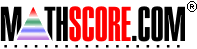Math Practice Online > free > lessons > Florida > 6th grade > Triangle Types

## Triangle Types

Determine whether a triangle is equilateral, isosceles, or scalene, and whether or not it is a right triangle.

 Sample Problems for Triangle Types Lesson for Triangle Types

### This topic aligns to the following state standards

Grade 5: Geo 1. Uses appropriate geometric vocabulary to describe properties and attributes of two- and three- dimensional figures (for example, obtuse and acute angles; radius; equilateral, scalene, and isosceles triangles.).
Grade 6: Meas 3. Classifies triangles according to the measurement of their angles and according to the length of their sides.